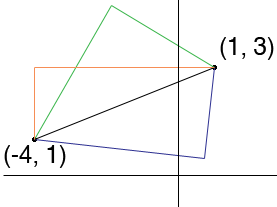SEARCH HOMEMath Central Quandaries & QueriesQuestion from Shubhomoy, a student: The co-ordinates of a hypotenuse are (1,3) and (-4,1). Find the equations of the perpendicular sides.Hi Shubhomoy,

There are many choices for the lines that form the other sides of the right triangle. Take any line through (1,3) that does not pass through (-4,1) and the line through (-4,1) that is perpendicular to the line you just choose.HarleyMath Central is supported by the University of Regina and The Pacific Institute for the Mathematical Sciences.